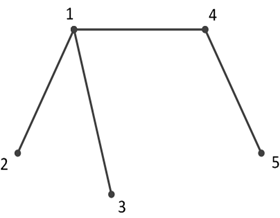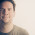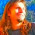## Thursday, April 23, 2015

### Fun and Games with CRV: Draw This Without Lifting Your Pencil

It's time for another installment in the "Fun and Games with CRV" series. I love doing these posts because there's something very engaging in modeling all sorts of problems as constraints. This time we're going to look at how to draw a barn without lifting our pencil from the paper and without doubling back. This wikiHow post shows us two ways how we could do that. Now lets see if we can write a solver that can find either of these solutions.

This problem is different from the other puzzle we've looked at before because it has a "time" component. We make moves one after the other and the order in which we make them limits what we can do in the next step. We know exactly how many moves we need to make; this is the number of edges the drawing has. This makes it easier to model, in comparison to more difficult problems where the number of steps is unknown.

While we are looking at how to draw a barn, this kind of puzzle is pretty widespread. Why not implement a more generic solver that can draw any type of figure? We can do this by splitting the solver part (the constraints) from the actual drawing we want to make. Such a drawing is merely a collection of edges, which connect two vertices each. These edges and vertices form a graph and what we want is to traverse it, by taking each edge exactly once. While for the drawing itself the direction of the edges doesn't matter (which is the start or the end vertex), it is important for how we draw the figure. We'll see how this impacts the solver later.

We'll encapsulate the task of modeling such a graph and the constraints needed to traverse it inside an own class. This drawer class (not the best of names, I admit) will provide a protected method that subclasses can call to define the edges of the drawing:

```virtual class drawer;
typedef struct {
rand vertex_t v1;
rand vertex_t v2;
} edge_t;

protected function void add_edge(vertex_t vertex1, vertex_t vertex2);
// ...
endfunction

// ...
endclass
```

My first try was to store all of the edges inside an array and add a constraint to try and randomly select one from the list:

```virtual class drawer;
local edge_t edges[\$];
local edge_t dummy_edge;

constraint choose_existing_edges {
dummy_edge inside { edges };
}
endclass
```

This would have been too easy and probably have made for too short a post. "Fortunately", the SystemVerilog LRM doesn't allow this kind of construct, restricting us to using only singular types with the inside operator (integers, bit vectors, enums, etc.). We'll need to store the connected vertices in some other way if we want to be able to solve this problem. The best way (I could think of) is an associative array of queues indexed by the vertex and containing a list of all other vertices it's connected to directly via edges:

```virtual class drawer;
typedef int unsigned vertex_t;
typedef vertex_t connections_t[\$];

local connections_t connections[vertex_t];
endclass
```

Let's look at an example:In the drawing above, this is what the connections matrix would contain:

```1 : { 2, 3, 4 }
2 : { 1 }
3 : { 1 }
4 : { 1, 5 }
5 : { 4 }```

```virtual class drawer;
protected function void add_edge(vertex_t vertex1, vertex_t vertex2);
if (connections.exists(vertex1) && vertex2 inside { connections[vertex1] })
\$fatal(0, "Connection %0d -> %0d already exists", vertex1, vertex2);

connect_vertices(vertex1, vertex2);
connect_vertices(vertex2, vertex1);

begin
edge_t e;
e.v1 = vertex1;
e.v2 = vertex2;
edges.push_back(e);
end
endfunction

local function void connect_vertices(vertex_t src, vertex_t dest);
if (connections.exists(src))
connections[src].push_back(dest);
else
connections[src] = '{ dest };
endfunction
endclass
```
The connect_vertices(...) function handles updating the array of connections. Even though the edges can't be used in constraints directly, we can still store them. The number of edges we add will be the number of steps we need to draw.
It's also a good idea to store a list of all the vertices we have in our drawing. This list we could easily extract from the connections array by taking all of its keys:
```virtual class drawer;
local vertex_t vertices[\$];

function void pre_randomize();
vertex_t v;
void'(connections.first(v));
do
vertices.push_back(v);
while (connections.next(v));
endfunction
endclass
```

At this point, it makes sense to try again and write a constraint that can select an edge from the ones we've added to the list. I wanted to try something fancy here:

```virtual class drawer;
constraint choose_existing_edge {
dummy_edge.v1 inside { vertices } &&
dummy_edge.v2 inside { connections[dummy_edge.v1] };
}
endclass
```

The idea was to choose the first vertex at random. The second vertex we could then choose from the list of vertices connected to the first one. In theory, this is great, but in practice, this constraint doesn't work because the index we are using for connections (dummy_edge.v1) is a random variable itself and this doesn't seem to be allowed. I'm not entirely sure if this is a simulator limitation or if the LRM forbids it.

We shouldn't give up on the idea just yet. With a bit of massaging, we can get it to compile. In the second constraint expression we can just loop over all entries inside connections and stop when we've reached the one corresponding to our chosen "starting" vertex:

```virtual class drawer;
constraint choose_existing_edges {
dummy_edge.v1 inside { vertices };

foreach (connections[v])
if (dummy_edge.v1 == v)
dummy_edge.v2 inside { connections[v] };
}
endclass
```

It's a little more code, but it does the job perfectly. Now we have a solid base to really start building our solver. We'll need to keep track of what edges we've already drawn and when. We'll store them in an array, where index 0 will be the first edge we drawn, index 1 the second and so on:

```virtual class drawer;
local rand edge_t edge_being_drawn[];
endclass
```

The first constraint we'll write is that each edge we draw actually exists in the drawing. We've already written such a constraint for one edge, so we'll just extend it to cover all of them:

```virtual class drawer;
constraint choose_existing_edges {
foreach (edge_being_drawn[i])
edge_being_drawn[i].v1 inside { vertices };

foreach (edge_being_drawn[i])
foreach (connections[v])
if (edge_being_drawn[i].v1 == v)
edge_being_drawn[i].v2 inside { connections[v] };
}
endclass
```

Just choosing edges that exist inside the figure isn't enough. We have to make sure that the edges are unique. Here I tried some more fanciness than the language affords. This constraint, though short and sweet, also doesn't compile:

```virtual class drawer;
constraint choose_unique_edges {
unique { edge_being_drawn };
}
endclass
```

As with the inside operator, the unique operator also only works on integral types. I tried to outsmart the compiler by declaring the struct as packed, but then it can't be declared as rand so that won't get us anywhere. As before, we're just going to have to throw some more code at the problem:

```virtual class drawer;
constraint choose_unique_edges {
foreach (edge_being_drawn[i])
foreach (edge_being_drawn[j])
if (i != j)
!(edge_being_drawn[i].v1 == edge_being_drawn[j].v1 &&
edge_being_drawn[i].v2 == edge_being_drawn[j].v2);

foreach (edge_being_drawn[i])
foreach (edge_being_drawn[j])
if (i != j)
!(edge_being_drawn[i].v1 == edge_being_drawn[j].v2 &&
edge_being_drawn[i].v2 == edge_being_drawn[j].v1);
}
endclass
```

As we already know from the previous post on array constraints we can replace a unique constraint with a double foreach. It's not enough to say that the starting or ending vertices be different. We also need to make sure that we're not backtracking. Going from vertex 1 to vertex 3 is the same edge when going from vertex 3 to vertex 1 (it's just the direction that's different).

One more constraint is missing to make the solution complete. We have to make sure that when going from one edge to another, the end vertex of the previous edge is the start vertex of the current edge. If we don't, then we're lifting our pencil from the paper. This is very easy to express:

```virtual class drawer;
constraint choose_connected_edges {
foreach (edge_being_drawn[i])
if (i > 0)
edge_being_drawn[i].v1 == edge_being_drawn[i - 1].v2;
}
endclass
```

Let's test it out by trying to draw a barn. Here's how the vertices are numbered:The barn_drawer class only needs to define the edges using the add_edge(...) function:

```class barn_drawer extends drawer;
function new();
endfunction
endclass
```

We just need to randomize an instance of barn_drawer and we should get the instructions we need to solve our puzzle. Sadly, things are never this easy. Immediately after putting all constraints together I got a constraint violation. After looking at the "choose" constraints over and over and hitting my head on the table, some time later I found what the problem was; it wasn't in my code. As in the first installment of the series, when solving Sudoku, a simulator bug reared its ugly head, but this time it wasn't as easy to pinpoint. I should probably consider moonlighting as a QA for an EDA company since I seem to draw such issues to me. Getting to the point, if we add this slight modification to the choose_unique_edges constraint it's going to make the clouds go away:

```virtual class drawer;
constraint choose_unique_edges {
// ...

foreach (edge_being_drawn[i])
foreach (edge_being_drawn[j])
// (Very) Possible bug in simulator
if (i == j - 1)
edge_being_drawn[i].v1 != edge_being_drawn[j].v2;
else if (j == i - 1)
edge_being_drawn[j].v1 != edge_being_drawn[i].v2;
else

// This condition should be enough by itself.
if (i != j)
!(edge_being_drawn[i].v1 == edge_being_drawn[j].v2 &&
edge_being_drawn[i].v2 == edge_being_drawn[j].v1);
}
endclass
```

And there we have it! Now we can solve any such "draw this without lifting your pencil" puzzle. We've also learned a bit more about the limitations of the SystemVerilog constraint language. I can't help thinking that the whole thing would have been much cleaner in e. I might try it out in the future just to see. If someone else does it, it would be nice to compare. In the meantime, you can find the code on SourceForge if you want to experiment drawing other figures.

1.Getting these to work sometimes feels like an exercise in self-torture. The most elegant constraints are rarely supported by either LRM or the tools. Even so, every time you do one of these, I always try to come up with my own solution. :)

I rarely (never? :) ) come up with something better, but I think this time I got an elegant one...

* We can represent required edges in a matrix m_edges[v1][v2], where m_edges[v1][v2] == 1 if there's an edge between v1 and v2.

* We can represent the drawing path using r_vertex[EDGES] array, where pencil starts on r_vertex, moves to r_vertex, etc.

* Then, the constraint is to say that for each r_vertex[i], r_vertex[i-1] pair, we must have m_edges[r_vertex[i]][r_vertex[i-1]]==1.

* The only thing missing now is the uniqueness. This is where I use a 3-D matrix r_edges[v1][v2][i] to represent whether step 'i' has drawn edge v1-v2 or not. With that available, we can then say that for each m_edges[v1][v2] == 1, we must have a one-hot {r_edges[v1][v2], r_edges[v2][v1]} (accounting for either direction).

Code: (it's hard to read, blogger doesn't allow code or pre tags in comments)

class drawer#(VERTICES=5,EDGES=8);

bit[VERTICES-1:0][VERTICES-1:0] m_edges;
rand byte unsigned r_vertex[EDGES:0];
rand bit[VERTICES-1:0][VERTICES-1:0][EDGES:0] r_edges;

constraint c_edges {
foreach(r_edges[v1, v2, i]) {
r_vertex[i] < VERTICES;
(i > 0) && (r_vertex[i] == v2) && (r_vertex[i-1] == v1) ->
r_edges[v1][v2][i-1] == 1 &&
m_edges[v1][v2] | m_edges[v2][v1] == 1;

m_edges[v1][v2] -> \$onehot({r_edges[v1][v2], r_edges[v2][v1]});
}
}
endclass

This works with VCS and Questa. VCS is a bit slow at it... It speeds up quite a bit if we explicitly set r_edges to 0 when they are not drawn on, but I was going for the least amount of constraint code :).

I think Incisive still doesn't support \$onehot in a constraint, but one could write a function to replace it.

2.Self-torture is probably a bit strong for it, but patience for sure. I wanted to dabble with using an adjacency matrix too for the solution, but I didn't want to go too deep into graph theory. Your solution is anyway pretty cool. It's interesting to see that one little extra constraint can speed up some solvers.

Regarding \$onehot, I had the exact same problem for N-Queens. I'd avoid it since as you say it's not supported by all sims, but a viable replacement is sum(). A handwritten function would create a unidirectional constraint and that would change the solution space. To use sum(), though we need to work with arrays of bits instead of vectors.

1.I have originally tried to use a 3-D array with .sum(), but managed to crash 2 of 3 simulators, with third complaining that it didn't support it yet. (That's probably where my self-torture comment came from :) ). So, that sent me the way of the 3-D vector instead.

Using a onehot() custom function would indeed change the solve order, but since it's essentially being used to "check" the answer at the end, it would still produce the correct answer here.